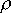QUESTION #360

# Why does the stream of water from a faucet become narrower as it falls?

This question refers to a rather well-known phenomenon that everyone can observe. Just turn on the water in your bathroom, and turn it down so it flows in a steady, non-turbulent manner. You can observe that the stream gets narrower as towards the sink, as it falls.

Why does this happen? The answer is really simple, only very simple principles need to be known to understand the behavior of the stream.

First, we assume 'steady' flow. By that, we mean that the flow does not change in time, the stream stays and behaves the same from one moment to the next. In this case, there is one simple observation:

The amount of water in any section of the stream stays the same.

This leads us to the next step:

The amount of water entering any section of the stream must equal the amount of water leaving that section at any time.

Since these observations are true for _any_ section of the stream, it can be generalized to the following statement:

The amount of water running through the cross section of the stream per unit time at any point is the same.

Now, how can we write the amount of water flowing through the cross section of the stream in terms of other quantities? It requires a little thought, but it is not too difficult.

First, it obviously depends on the cross section. The more cross section there is, the more flow there will be per unit time. (In analogy with a highway, the wider the highway, the more traffic it can accommodate.)

Second, it also depends on the _speed_ of the flow. The more speed, the more flow. (Again, in analogy with a highway, the faster the cars are traveling, the more traffic can run through.)

Lastly, the last dependence is on the density of the fluid -- in general it can change from point to point within the fluid (although it is constant to a very good approximation for your tap water).

So, we arrive at the following equation:1 * v1 * A1 =2 * v2 * A2

Where1 and2 are densities at the two cross sections in question, v1 and v2 are the velocities, and A1 and A2 are the areas.

As mentioned before, the density of water is pretty much a constant. So we have1 =2, which leaves us with:

v1 * A1 = v2 * A2

Now, our observation suggests that, at lower points in the stream, the cross section is smaller (i.e. the stream is narrower). For the last equation to hold, it must be true that the velocity at the lower part of the stream must be _higher_ (so that the product remains the same). How can that be? The answer is simple: Gravity. Like all objects, a stream of water also accelerates as it falls. Since the density of water is a constant, and water tends to hold together (cohesion), the only thing that can happen is the narrowing of the stream -- which is exactly what happens.
Answered by: Yasar Safkan, Ph.D. M.I.T., Software Engineer, Istanbul, Turkey